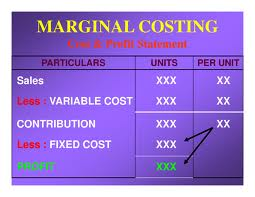Accounting

# What is Meant by Marginal Costing?What is meant by Marginal Costing?

The marginal cost of an additional unit of output is the cost of the additional inputs needed to produce that output.  More formally, the marginal cost is the derivative of total production costs with respect to the level of output.Marginal cost is the cost to a firm of producing one extra unit of product.

Marginal cost and average cost can differ greatly.  For example, suppose it costs \$1000 to produce 100 units and \$1020 to produce 101 units.  The average cost per unit is \$10, but the marginal cost of the 101st unit is \$20

The Econ Model applications Perfect Competition and Monopoly emphasize the roles of average cost and marginal cost curves.  The short movieDerive a Supply Curve (40 seconds) shows an excerpt from the Perfect Competition presentation that derives a supply curve from profit maximizing behavior and a marginal cost curve.  A firm achieves its maximum possible total profit when MR = MC (ie: when marginal profit = 0, which means that producing and selling one extra unit will cost \$x and will yield revenue of \$x too). Before the point where MR = MC, total profit is rising. After that point, total profit is declining (but there are not necessarily losses).

When making decisions regarding production increases or decreases, a firm should base its thinking on marginal costs and revenues rather than on total or average ones because it is the “marginal” side of the picture that will change when output is changed.